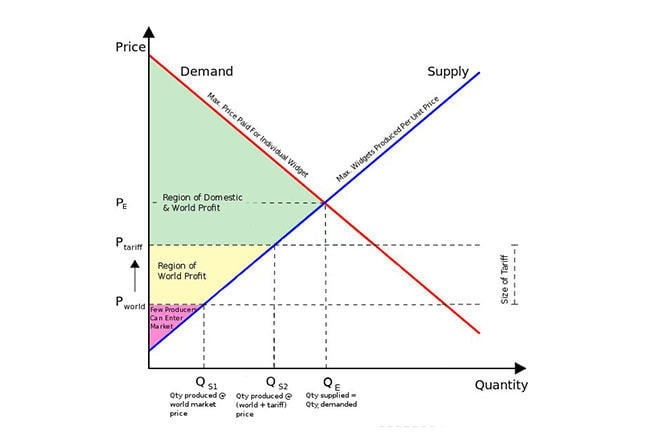## Want to keep learning?

This content is taken from the UNSW Sydney's online course, Maths for Humans: Linear and Quadratic Relations. Join the course to learn more.
2.6

## UNSW SydneyHow much should you charge your customers for cupcakes?

# Supply and demand for Aunt Florrie's cupcakes

Let’s say you have a cafe in a small town, and you decide to sell some of your Aunt Florrie’s cupcakes. But you face an important decision: how to set a price? If you price them too high, you won’t sell a lot, but if you price them too low, you won’t make much profit.

In this step, we will

• learn about supply and demand curves, or lines

• find the sweet spot when it comes to pricing Aunt Florrie’s cupcakes.

## A touch of microeconomics

Microeconomics is the study of the economic decisions of individuals and companies, and in particular their behaviours in markets where goods and services are sold. In microeconomics, we are particularly interested in the relation between the quantity $$\normalsize{Q}$$ of a good (for example cupcakes) and its price $$\normalsize{P}$$.

Let’s say you experiment with different pricing structures: you quickly find out that the higher your price for cupcakes, the fewer cupcakes customers buy. Perhaps, after more experimentation, you find that Demand is linearly related to the Price, as shown by these figures.

Price ($$\normalsize{P}$$) Quantity ($$\normalsize{Q}$$)
$4.50 8$4.00 12
$3.50 16$3.00 20
$2.50 24$2.00 28

Q1 (E): What is the slope of the line passing through these points, with $$Q$$ as the horizontal axis and $$P$$ as the vertical axis?

## Demand curve

We can graphically represent this data with a graph of a Demand Curve, which in this case is a line. In real life, this curve is not going to be exactly a line, but economists, like the rest of us, prefer to make simplifying assumptions so that things are easier to understand. One advantage in dealing with lines is that they have simple linear equations. In our case the Demand curve has equation:

$\Large{P=\frac{11}{2}-\frac{1}{8}Q}$Q2 (E): How high can you jack up the price of cupcakes before there are no sales?

## Supply curve

Somewhat more subtle is that the supplier of the good also is influenced by its Price, but in the opposite direction of the consumer. Aunt Florrie is not that keen on making more than a dozen or perhaps two cupcakes a week, but if she gets a better price for them, her motivation for making more increases. The following values describe Florrie’s Supply Curve (in this case a line) which describes how much she is willing to make at a given price level.

Price ($$\normalsize{P}$$) Quantity ($$\normalsize{Q}$$)
$4.50 38$4.00 32
$3.50 26$3.00 20
$2.50 14$2.00 8

This Supply line has equation

$\Large{P=\frac{1}{12}Q+\frac{4}{3}}.$Q3 (E): If you offer Aunt Florrie one dollar, will she make you a cupcake?

## An equilibrium point

Economists are very interested in equilibrium points; in this case where the Demand Curve and the Supply Curve meet. From the tables, we can see this is when $$\normalsize{P=\3}$$ and $$\normalsize{Q=20}$$. If the price gets any higher than that, although we will have more cupcakes available, we won’t have enough demand for people to buy them. If the price is lower, there will be more demand, but not enough cupcakes, since Aunt Florrie will rather spend her time bowling with her friends.

So the point $$\normalsize{[20,3]}$$ is an equilibrium point—the sweet spot for this particular economic story. We could also find this point by solving the two equations for the lines:

$\Large{P=\frac{11}{2}-\frac{1}{8}Q}$ $\Large{P=\frac{4}{3}+\frac{1}{12}Q}$What do you think are the pros and cons of this kind of analysis? Share your answers in the discussion.

A1. Using the points $$[20,3]$$ and $$[12,4]$$ the slope is

${\Large \frac{\Delta P}{\Delta Q} = \frac{4 - 3}{12 - 20} = -\frac{1}{8}}.$

A2. You will sell exactly one cupcake when $$P=\frac{43}{8}$$. Any price bigger than this will result in no sales.

A3. When $$\normalsize P=1$$ on the supply line, $${\normalsize 1 =\frac{1}{12}Q+\frac{4}{3} }$$, so that $$\normalsize Q = -4$$. This is telling us that Aunt Florrie is unwilling to make any cupcakes at this price. In fact the minimum price for which she will even begin to consider making cupcakes is the $$\normalsize P$$-intercept of the Supply line, which is $$\normalsize P=4/3$$.